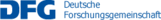## C08 – Multilinear estimates in geometric Fourier analysis

The goal of this project is to study Fourier analytic questions relating to symmetries, in particular rotational and scaling symmetry, of Euclidean space. Such symmetry adds a specific geometric flavor to of the analytic question at hand. The main vehicle proposed to address these questions are multilinear estimates, which can take the form of paraproduct type estimates if scaling is one of the symmetries of the problem.

One example of a geometric Fourier analytic question of interest is the sharp constant in the Tomas–Stein Fourier extension inequality in two dimensions. The Fourier extension map in two dimensions maps an L^2 function on the circle to an L^6 function on the plane. Thanks to the even integer exponent 6, this map can be viewed as a multilinear operator. Multilinear techniques have been employed by D. Foschi for the corresponding easier problem in three dimensions. For the problem in two dimensions partial progress was achieved by the PI in collaboration with Carneiro, Foschi, Oliveira e Silva. Analogous sharp restriction and extension problems can be asked for other conical sections than the sphere and are tantamount to Strichartz estimates that are ubiquitous in dispersive PDE.

A second example of geometric Fourier analytic questions concerns singular variants of Brascamp–Lieb inequalities, for example determinant functionals arising from a Brascamp–Lieb type integral together with a singular kernel that is a power of a determinant. Multilinear estimates for such integrals are used as a vehicle to study Fourier extension phenomena and also have become an object of study in their own right. Encouraging progress was made for a two dimensional singular Brascamp–Lieb
inequality, but the method there relies on very strong uniform for a more singular object and is restricted to the particular two dimensional example. A goal is to find a more flexible paraproduct type proof with the goal of generalizing the estimate to higher dimensions.

A new tool to study multilinear estimates was created by the PI in collaboration with Do, where a novel integration theory was created for outer measure spaces which need not possess many Caratheodory measurable sets. This outer integration theory is very adapted to scale invariant questions such as raised here. In particular, outer measures explain paraproduct estimates. This theory is implicitly used in the study of determinant functionals and expected to play a role in any deeper understanding of these functionals.

A third example of geometric analytic questions concerns estimates for directional versions of standard operators in harmonic analysis such as the directional maximal operator and the directional Hilbert transform. These operators relate to rotation symmetry via an action of the rotation group on the set of directions, and they also exhibit invariance under scaling.

One motivation for this project is to refine fundamental tools in harmonic analysis which
play a role in many other projects of this CRC 1060.

Name Institute Location Phone
Thiele, ChristophMIEn60/3.01062254thiele@math.uni-bonn.de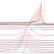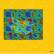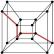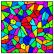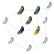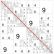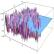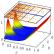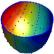## Contact

Coordinator: Prof. Dr. Stefan Müller